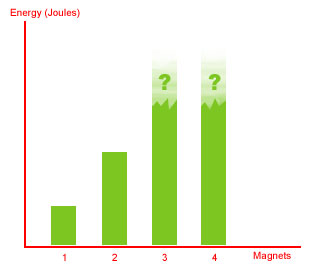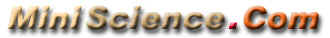## The Gauss Rifle: A Magnetic Linear Accelerator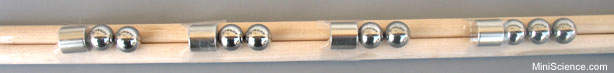The Gauss Rifle also known as the Gaussian gun is a very simple experiment that uses a magnetic chain reaction to launch a steel marble at a target at high speed. This educational and amusing project is very simple to build (it only takes a few minutes), is is very simple to understand and explain, and yet fascinating to watch and to use. The movie in the right shows a sample of the gauss rifle in action. In the beginning, a steel ball starts rolling towards a magnet taped to a plastic rail. As soon as the rolling ball hits the magnet, another ball in the opposite side is launched and leaves the device at a very high speed.
 The materials are simple. We need a straight path or rail in which balls can roll easily. Any piece of wood, plastic, aluminum or brass with a groove will work. A wooden ruler that has a groove in the top is a good choice because it is easy to find around the house or at school or at a local stationery store. Another option is using two wood dowels or rods or copper pipes placed side by side. If you are making a rail using two wood dowels, temporarily connect them to each other using clear adhesive tape, then use wood glue to glue them to each other from one side. Use a paper towel to remove excess glue and allow the glue dry for a few hours. When the glue is dry, you can remove the adhesive tapes. Use the unglued side as the rail.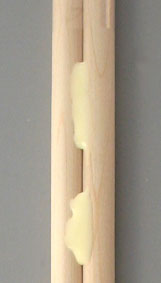We need about four strong magnets. I suggest super strong cylindrical magnets (such as N35.500.500) with the same diameter as the steel balls. Cylindrical magnets can easily sit in the grove and can easily be secured in place.

We need some sticky tape. Again, almost any kind will do. Here we use Scotch brand transparent tape, but vinyl electrical tape works just as well.

We will also need nine steel balls, with a diameter that is a close match to the diameter of the magnets. We use 1/2 inch diameter nickel plated steel balls.

 The only tool you will need is a sharp knife for trimming the tapes. (Optional). If you want to trim the tapes, be careful, since the knife will be strongly attracted to the magnets.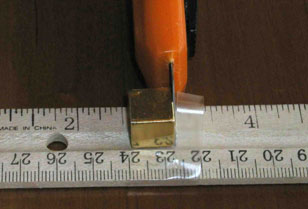Procedure:

Start by taping the first magnet to the rail at the 2.5 inch mark. The distance is somewhat arbitrary. Just keep in mind that we are using a short ruler and all four magnets should go on a one foot rail. Feel free to experiment with the spacing later. If you are not using a ruler as the rail, then you can estimate the distances or measure them to ensure you are using the recommended distance.

It is very important that you keep the magnets from jumping together. They are made of a brittle sintered material that shatters like a ceramic. Tape the ruler to the table temporarily, so that it doesn't jump up to the next magnet as you tape the second magnet to the ruler.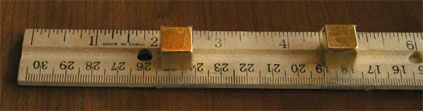Continue taping the magnets to the ruler, leaving 2.5 inches between the magnets.

When all four magnets are taped to the ruler, it is time to load the gauss rifle with the balls.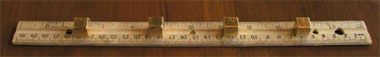To the right of each magnet, place two steel balls. Arrange a target to the right of the device, so the ball does not roll down the street and get lost.

To fire the gauss rifle, set a steel ball in the groove to the left of the leftmost magnet. Let the ball go. If it is close enough to the magnet, it will start rolling by itself, and hit the magnet.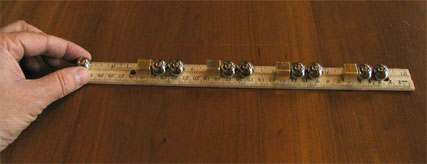When the gauss rifle fires, it will happen too fast to see. The ball on the right will shoot away from the gun, and hit the target with considerable force. Our one foot long version is designed so the speed is not enough to hurt someone, and you can use your hand or foot as a target.

## How does it do that?

When you release the first ball, it is attracted to the first magnet. It hits the magnet with a respectable amount of force, and a kinetic energy we will call "1 unit".

The kinetic energy of the ball is transferred to the magnet, and then to the ball that is touching it on the right, and then to the ball that is touching that one. This transfer of kinetic energy is familiar to billiards players -- when the cue ball hits another ball, the cue ball stops and the other ball speeds off.

The third ball is now moving with a kinetic energy of 1 unit. But it is moving towards the second magnet. It picks up speed as the second magnet pulls it closer. When it hits the second magnet, it is moving nearly twice as fast as the first ball.

The third ball hits the magnet, and the fifth ball starts to move with a kinetic energy of 2 units. It speeds up as it nears the third magnet, and hits with of 3 units of kinetic energy. This causes the seventh ball to speed off towards the last magnet. As it gets drawn to the last magnet, it speeds up to 4 units of kinetic energy.

The kinetic energy is now transferred to the last ball, which speeds off at 4 units, to hit the target.

## Another way of looking at the mechanism

When the device is all set up and ready to be triggered, we can see that there are four balls that are touching their magnets. These balls are at what physicists call the "ground state". It takes energy to move them away from the magnets.

 But each of these balls has another ball touching it. These second balls are not at the ground state. They are each 1/2 of an inch from a magnet. They are easier to move than the balls that are touching the magnet. If we were to take a ball that was touching a magnet, and pull it away from the magnet until it was 1/2 of an inch away, we would be adding energy to the ball. The ball would be pulling towards the magnet with some considerable force. We could get the energy back by letting the ball go. After the gauss rifle has fired, the situation is different. Now each of the balls is touching a magnet. There is one ball on each side of each magnet. Each ball is in its ground state, and has given up the energy that was stored by being 1/2 of an inch from a magnet. That energy has gone into the last ball, which uses it to destroy the target.

## Speed and kinetic energy

The kinetic energy of an object is defined as half its mass times the square of its velocity. As each magnet pulls on a ball, it adds kinetic energy to the ball linearly.

But the speed does not add up linearly. If we have 4 magnets, the kinetic energy is 4, but the speed goes up as the square root of the kinetic energy. As we add more magnets, the speed goes up by a smaller amount each time. But the distance the ball will roll, and the damage it causes to what it hits, is a function of the kinetic energy, and thus a function of how many magnets we use.

We can keep scaling up the gun until the kinetic energy gets so high that the last magnet is shattered by the impact. After that, adding more magnets will not do much good.

 If you don't have this kit, you can order it now! It is available both as a single pack and class pack. Kit content may be different from the images shown in this page. The strength of magnets in this kit is 35 MGOe (Mega Gauss Oersted)= 3500 Tesla.Secure Online Store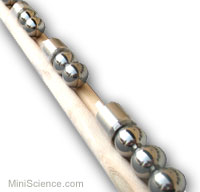Magnetic linear accelerator Science Project:

If you are doing this science project, you may use the following ideas for the question, variables, hypothesis and experiments.

Question: How does the number of magnets affect the kinetic energy of the gauss rifle?

Variables:

Independent variable is the number of magnets, Dependent variable is the kinetic energy.

Hypothesis:

Each additional magnet will double the kinetic energy compare to the previous magnet.

Procedure:

Place your magnetic accelerator or Gauss Rifle horizontally on the edge of a table about 100 cm tall. Select the location of the table where you have at least 10 feet open space for the ejected ball.

 Load it with one magnet, two balls in front of it and a third ball (call it trigger ball) rolling toward the magnet from the back. Measure the ground distance the ball is ejected and write it in your data table.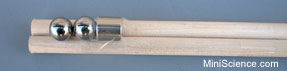Repeat this with 2, 3 and 4 magnets and record the ejection distance for each number of magnets.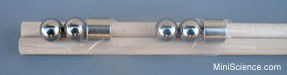Your data table may look like this:

Ejection distance data for Magnetic linear accelerator

 Number of accelerating magnets Ejection Distance (Meter) 1 2 3 4

Use the ejection distance to calculate the initial kinetic energy of the projectile. Calculating the kinetic energy of a projectile is fairly straight forward using the following equation:

KE=1/2 m * v2 or KE= 1/2 m * (d/t)2

where m is the mass of the projectile in kilograms and v is the velocity in meters per second and KE is energy in Joules. In the second formula d is the ejection distance (in meters) and t is the travel time (in seconds).

The m for 1/2" steel ball is 8.4 grams or 0.0084 Kilogram.  t is 0.45 seconds (This is the time it takes for any object to fall from one meter elevation, disregarding the air friction).

So by knowing m = 0.0084 kg and  t = 0.45 seconds you can calculate the kinetic energy of a projectile that falls at d = 2.7 meters away from the table.

KE = 1/2 * 0.0084 * (2.7 / 0.45)2 = 0.1512 Joules

Kinetic Energy of our Magnetic Linear Accelerator

 Number of accelerating magnets Ejection Distance (Meter) Kinetic Energy (Joule) 1 0.92 0.0175 2 1.32 0.0361 3 .... 4 ....

Make a graph:

Use the above table to make a bar graph for your results. Use one bar for each number of magnets from 1 to 4. The height of each bar will represent the kinetic energy you calculated with that number of magnets.
 This is a sample graph, but it is not complete. You must make a graph using your own data. Also please verify the accuracy of the calculations.